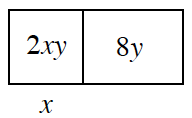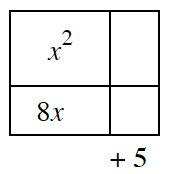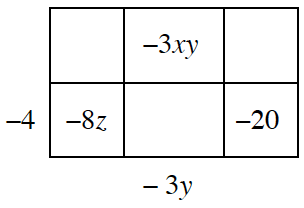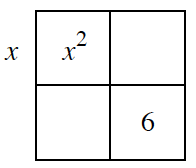Home > INT2 > Chapter 2 > Lesson 2.3.1 > Problem2-79

2-79.

Copy each of the area models below and fill in the missing dimensions and areas. Then write the entire area as a product equivalent to the area as a sum. Homework Help ✎

 a.Hint (a):Try to find a way to factor what is inside of the boxes, then it is possible to write the area as a product of its sum.Answer (a):$2xy+8y=2y(x+4)$ b.Answer (b):$(x+8)(x+5)=x^2+13x+40$ c.Answer (c):$(x−4)(2z−3y+5)=2xz-3xy+5x-8z+12y−20$. d.Answer (d):Multiple answers are possible.